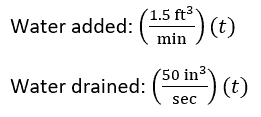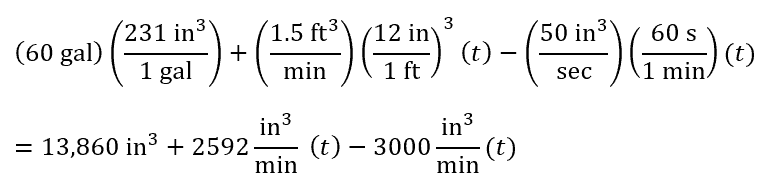Return to Blog Homepage

# PCAT Quantitative Reasoning – Bathtub Filling Rate Word Problem

A bathtub contains 60 gallons of water. The bathtub is being filled at a rate of 1.5 ft3 per minute. At the same time, water drains through a pipe at a rate of 50 in3 per second. At what point will the bathtub be empty? (1 gal = 231 in3)

1. 5 min
2. 18 min
3. 34 min
4. 408 min
##### Explanation

C is correct. The bathtub will be empty once the total volume of water in the bathtub equals zero. We can write an equation for the total volume of water in the bathtub at any given time by adding the initial volume of water and the volume of water added, and subtracting the volume of water drained. The amounts of water being added and drained are expressed in the word problem as rates. To convert into volume, we can multiple the rate by time (t) such that the unit for time cancels out:Therefore, the total volume held by the bathtub at any given time is:. To add these together, we need each term to have the same units. Let’s convert each term so that each uses the units in3 and min. Don’t forget to multiply the conversion factor from feet to inches three times to convert from ft3 to in3:Now let’s set this equation equal to 0 and solve for t to find the moment at which the bathtub is empty: